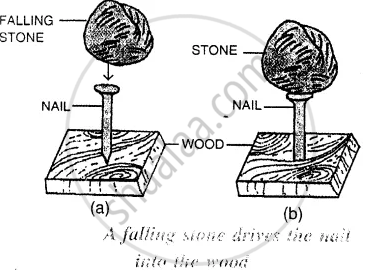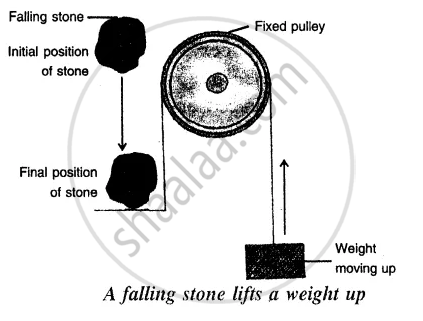ICSE Class 7CISCE
Share

# Give One Example to Show the Conversion of Potential Energy to Kinetic Energy When Put in Use. - ICSE Class 7 - Physics

ConceptDifferent Forms of Energy

#### Question

Give one example to show the conversion of potential energy to kinetic energy when put in use.

#### Solution

The example to show the conversion of potential energy to kinetic energy when put in use is :
A stone at a height has the potential energy due to its lifted or raised position. In the figure below when the stone is dropped from that position, it begins to fall. The falling stone has the kinetic energy. Thus, the potential energy stored in the stone in its raised position changes into the kinetic energy when the stone is falling. This kinetic energy does work on the nail as the stone strikes the nail arid makes the nail to move into the wood.Similarly, in the figure below the potential energy possessed by the stone at a height changes into its kinetic energy when it falls, The kinetic energy of the falling stone does work in raising the weight upwards.Is there an error in this question or solution?

#### APPEARS IN

Solution Give One Example to Show the Conversion of Potential Energy to Kinetic Energy When Put in Use. Concept: Different Forms of Energy.
S